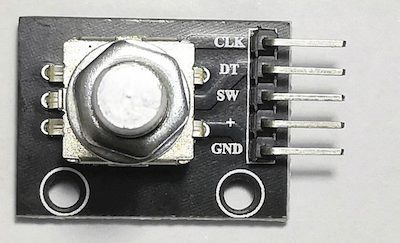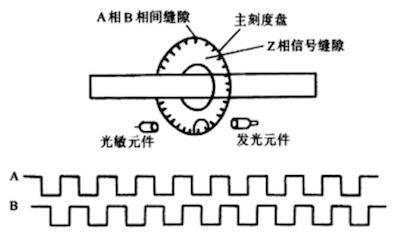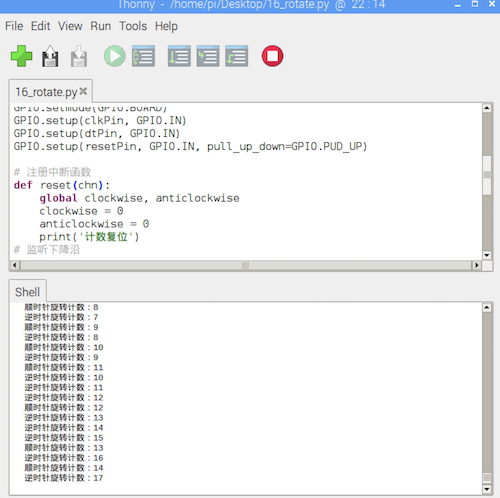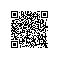# 一起玩转树莓派（16）——旋转编码传感器

旋转编码传感器是一种速度位移传感器。其通过旋钮的旋转来带动内部的光栅盘旋转，光栅盘上会预置很多狭缝，光栅盘的转动导致经过狭缝的光线产生脉冲变化，此信号经过后继电路的处理后，输出成脉冲信号。最终，我们可以通过信号引脚的输出来获取旋钮的旋转动作。

本次实验，使用的旋转编码传感器如下图所示：下面，我们来连接树莓派进行实验，接线如下：

VCC+5V
GNDGND
CLKGPIO17 （BCM编码）
DTGPIO18 （BCM编码）
SWGPIO19 （BCM编码）

#coding:utf-8

import RPi.GPIO as GPIO
import time

# 定义引脚
resetPin = 35 # BCM编码为19的GPIO 物理编码为35
clkPin = 11
dtPin = 12

# 计数变量
clockwise = 0
anticlockwise = 0

# 进行引脚初始化
GPIO.setmode(GPIO.BOARD)
GPIO.setup(clkPin, GPIO.IN)
GPIO.setup(dtPin, GPIO.IN)
GPIO.setup(resetPin, GPIO.IN, pull_up_down=GPIO.PUD_UP)

# 注册中断函数
def reset(channel):
global clockwise, anticlockwise
clockwise = 0
anticlockwise = 0
print('计数复位')
# 监听下降沿

# 开启主循环
while True:
lastDT = GPIO.input(dtPin)
# 高电平 没有发生旋转动作
while GPIO.input(clkPin):
pass
# 开始旋转
currentDT = GPIO.input(dtPin)
if (lastDT == 1) and (currentDT == 0):
# 顺时针
clockwise += 1
print('顺时针旋转计数：%d'%(clockwise))
if (lastDT == 0) and (currentDT == 1):
# 逆时针
anticlockwise += 1
print('逆时针旋转计数：%d'%(anticlockwise))IoT使用钉钉扫一扫加入圈子
+ 订阅